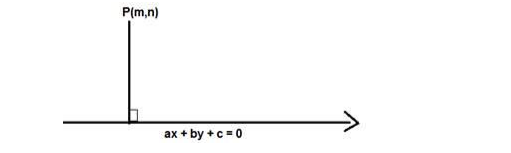# Find the distance of the pointQuestion:

Find the distance of the point (4, 2) from the line joining the points (4, 1) and (2, 3)

Solution:

Given: Point (4,2) and the line joining the points (4, 1) and (2, 3)

To find: The distance of the point $(4,2)$ from the line joining the points $(4,1)$ and $(2,3)$

Formula used: The equation of the line joining the points $\left(x_{1}, y_{1}\right)$ and $\left(x_{2}, y_{2}\right)$ is given by

$\frac{y-y_{1}}{y_{2}-y_{1}}=\frac{x-x_{1}}{x_{2}-x_{1}}$

Here $x_{1}=4 y_{1}=1$ and $x_{2}=2 y_{2}=3$

$\frac{y-1}{x-4}=\frac{3-1}{2-4}=\frac{2}{-2}=-1$

$y-1=-x+4$

$x+y-5=0$

The equation of the line is $x+y-5=0$

Formula used: We know that the distance between a point $P(m, n)$ and a line $a x+b y+$ $c=0$ is given by,

$D=\frac{|a m+b n+c|}{\sqrt{a^{2}+b^{2}}}$The equation of the line is $x+y-5=0$

Here $m=4$ and $n=2, a=1, b=1, c=-5$

$D=\frac{|1(4)+1(2)-5|}{\sqrt{1^{2}+1^{2}}}$

$D=\frac{|4+2-5|}{\sqrt{1+1}}=\frac{|6-5|}{\sqrt{2}}=\frac{|1|}{\sqrt{2}}$

$D=\frac{1}{\sqrt{2}}=\frac{\sqrt{2}}{2}$

The distance of the point $(4,2)$ from the line joining the points $(4,1)$ and $(2,3)$ is $\frac{\sqrt{2}}{2}$ units